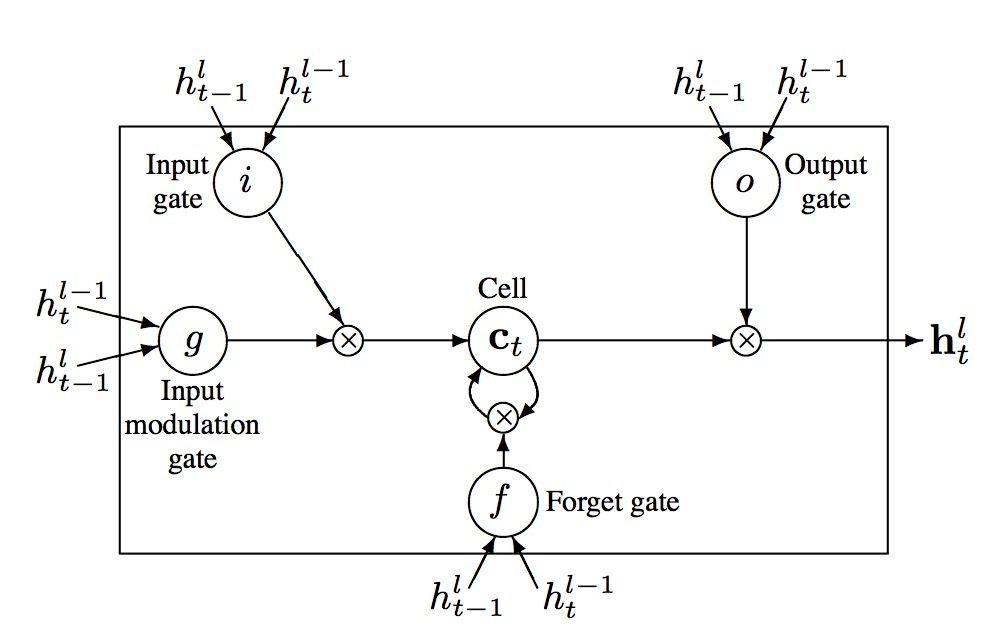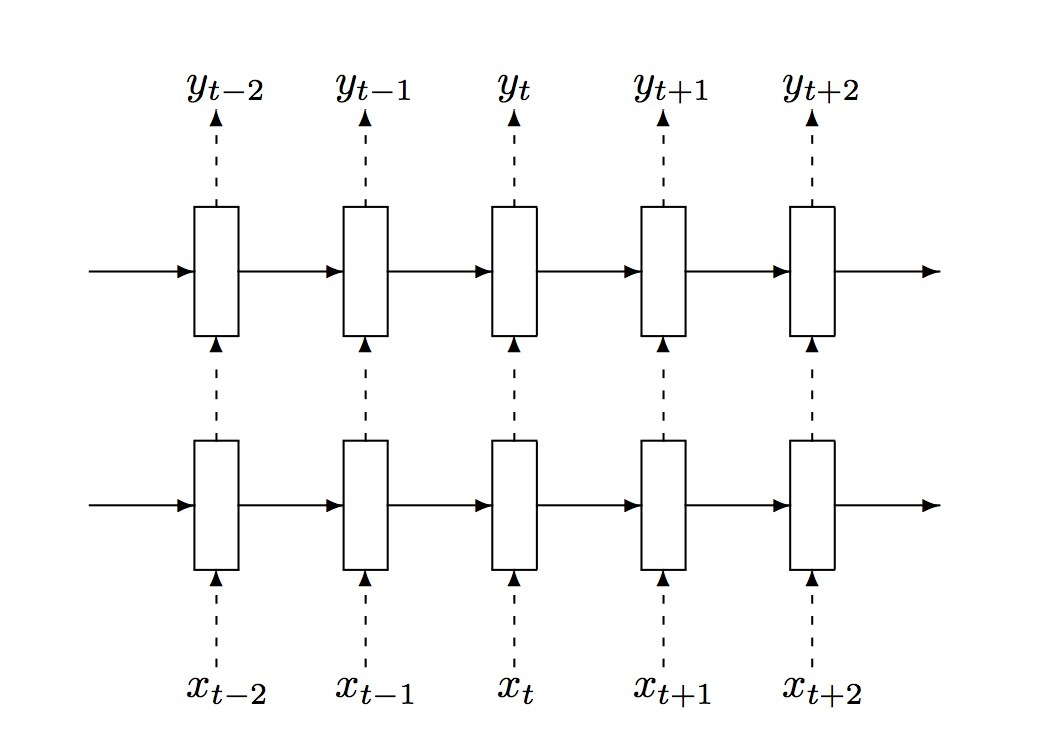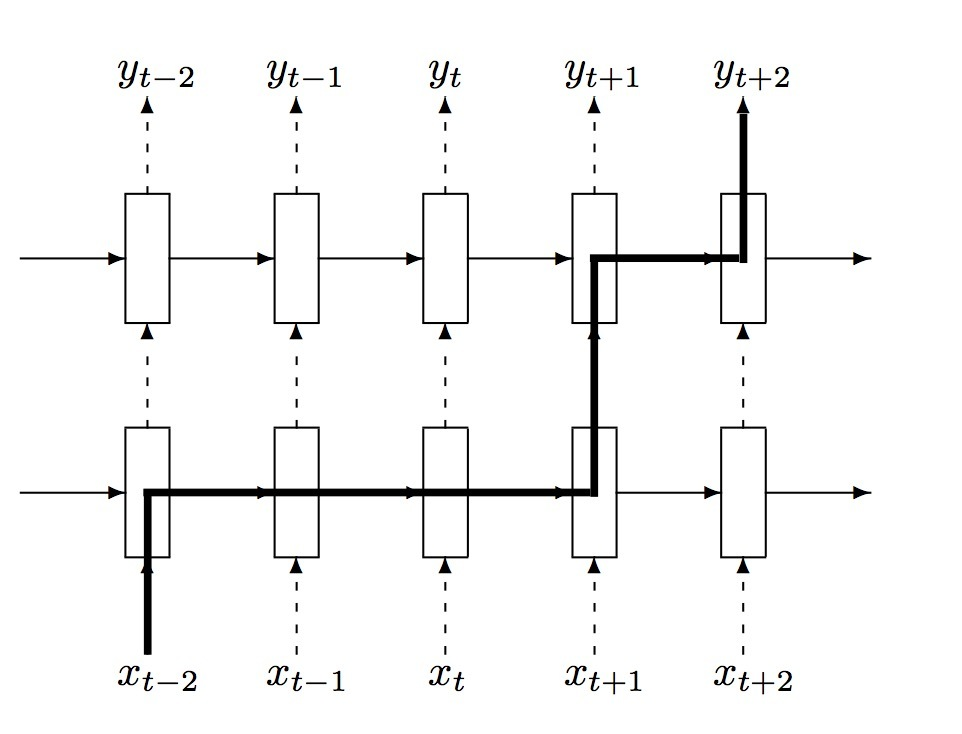1.介绍

RNN是神经序列模型，并在多个重要任务上达到了state-of-the-art的效果。我们知道，神经网络的成功应用需要好的正则化（regularization）。不幸的是，对于前馈神经网络最重要的regularzation：dropout(Srivastava 2013)，却不能与RNNs有效运行。因而，RNNs的实际应用经常使用很小的模型，因为大的RNNs会趋向于overfit。已经存在的regularization方法对于RNNs的相对提升较小。在本文中，我们展示了正确使用dropout时可以极大减小在LSTMs中的overfitting，并在三个不同问题上进行了evaluate。

3.LSTM cells RNN的正则化

• $h_t^l \in R^n$是在timestep t时在layer l上的一个hidden state。
• $T_{n,m}: R^n \rightarrow R^m$是一个仿射变换（Wx+b, 对于一些W和b）。
• $\odot$是element-wise乘法，
• $h_t^0$是一个在timestep t上的input word vector。

3.1 LSTM units

RNN的动态性可以使用从前一hidden states到当前hidden states上的确定转换（deterministic transitions）来描述。deterministic state transition是一个函数：

$RNN: h_t^{l-1}, h_{t-1}^l \rightarrow h_t^l$

$h_t^l = f(T_{n,n} h_t^{l-1} + T_{n,n} h_{t-1}^l), where \ f \in \lbrace sigm, tanh \rbrace$

LSTM具有复杂的动态性，使得它对于可以轻易地“记住（memorize）” 一段时间内的信息。“long term” memory被存储在memory cells $c_t^l \in R^n$的一个vector中。尽管许多LSTM的架构在它们的连接结构和激活函数上有所区别，所有的LSTM结构都具有显式的memory cells来存储长期的信息。LSTM可以决定是否overwrite memory cell，或者检索它，或者在下一time step上继续保留它。LSTM的结构如下：

$LSTM: h_t^{l-1}, h_{t-1}^l, c_{t-1}^l \rightarrow h_t^l,c_t^l \\ \begin{pmatrix} i \\ j \\ o \\ g \end{pmatrix} = \begin{pmatrix} sigm \\ sigm \\ sigm \\ tanh \end{pmatrix} T_{2n, 4n} \begin{pmatrix} h_t^{l-1} \\ h_{t-1}^l \end{pmatrix} \\ c_t^l = f \odot c_{t-1}^l + i \odot g \\ h_t^l = o \odot tanh(c_t^l)$3.2 使用dropout正则化$\begin{pmatrix} i \\ j \\ o \\ g \end{pmatrix} = \begin{pmatrix} sigm \\ sigm \\ sigm \\ tanh \end{pmatrix} T_{2n, 4n} \begin{pmatrix} D(h_t^{l-1}) \\ h_{t-1}^l \end{pmatrix} \\ c_t^l = f \odot c_{t-1}^l + i \odot g \\ h_t^l = o \odot tanh(c_t^l)$参考

Updated on

STAR算法介绍

PLE介绍

Published on March 04, 2021

DTS介绍

Published on January 02, 2021